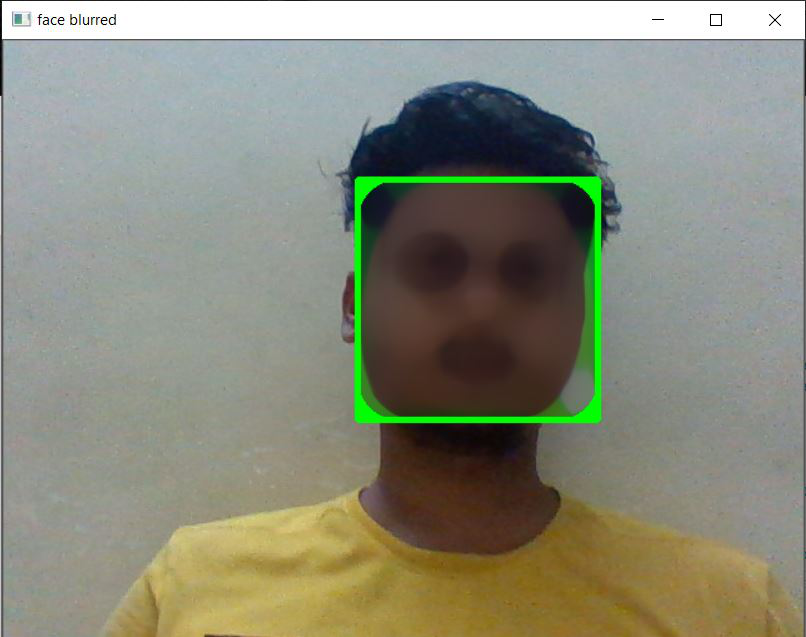# Blur and anonymize faces with OpenCV and Python

• Last Updated : 12 Nov, 2021

In this article, we are going to see how to Blur and anonymize faces with OpenCV and Python.

Attention geek! Strengthen your foundations with the Python Programming Foundation Course and learn the basics.

To begin with, your interview preparations Enhance your Data Structures concepts with the Python DS Course. And to begin with your Machine Learning Journey, join the Machine Learning - Basic Level Course

## Approach

• Firstly, we use a built face detection algorithm, to detect the face from a real-time video or from an image. Here, we will be using the cascade classifier method to detect a face from real-time video(using a webcam).
• Then, the frames from the real-time video are read. The latest frame is stored and is converted into grayscale, to understand the features in a better manner.
• Now, to make the output, aesthetically pleasing, we will make a color-bordered rectangle around the detected face. But, we want the detected face to be blurred, so we use the medianBlur function to do the same, and mention the area, up to which the face should be blurred.
• And, now we want to show the blurred face, the frame which was read using imshow function, and we want it to be shown, till we press a key.

## Stepwise Implementation:

Step 1: Importing the Face Detecting Algorithm, called Cascade Classifier.

## Python3

 `import` `cv2`` ` `# to detect the face of the human``cascade ``=` `cv2.CascadeClassifier(``"haarcascade_frontalface_default.xml"``)`

Step 2: Capturing the frames from the video, in order to detect the face from the frame

## Python3

 `video_capture ``=` `cv2.VideoCapture(``0``)``while` `True``:``   ` `    ``# capture the latest frame from the video``    ``check, frame ``=` `video_capture.read()`

Step 3: The captured frame being changed to grayscale.

## Python3

 `# convert the frame into grayscale(shades of black & white)``gray_image ``=` `cv2.cvtColor(frame, cv2.COLOR_BGR2GRAY)``face ``=` `cascade.detectMultiScale(gray_image,``                                ``scaleFactor``=``2.0``,``                                ``minNeighbors``=``4``)`

Step 4: Drawing a colored rectangle around the detected face.

## Python3

 `for` `x, y, w, h ``in` `face:`` ` `    ``# draw a border around the detected face.``    ``# (here border color = green, and thickness = 3)``    ``image ``=` `cv2.rectangle(frame, (x, y),``                          ``(x``+``w, y``+``h), ``                          ``(``0``, ``255``, ``0``), ``3``)`

Step 5: Blur the portion within the rectangle(containing the detected face).

## Python3

 `# blur the face which is in the rectangle``image[y:y``+``h, x:x``+``w] ``=` `cv2.medianBlur(image[y:y``+``h, x:x``+``w], ``35``)`

Step 6: Show the final output, i.e. the detected face(within the rectangle) is blurred.

## Python3

 `# show the blurred face in the video``cv2.imshow(``'face blurred'``, frame)``key ``=` `cv2.waitKey(``1``)`

Below is the complete implementation:

## Python3

 `import` `cv2`` ` `# to detect the face of the human``cascade ``=` `cv2.CascadeClassifier(``"haarcascade_frontalface_default.xml"``)`` ` `# VideoCapture is a function, to capture``# video from the camera attached to system``# You can pass either 0 or 1``# 0 for laptop webcam``# 1 for external webcam``video_capture ``=` `cv2.VideoCapture(``0``)`` ` `# a while loop to run infinite times,``# to capture infinite number of frames for video``# because a video is a combination of frames``while` `True``:``   ` `    ``# capture the latest frame from the video``    ``check, frame ``=` `video_capture.read()`` ` `    ``# convert the frame into grayscale(shades of black & white)``    ``gray_image ``=` `cv2.cvtColor(frame, cv2.COLOR_BGR2GRAY)`` ` `    ``# detect multiple faces in a captured frame``    ``# scaleFactor: Parameter specify how much the``    ``# image sizeis reduced at each image scale.``    ``# minNeighbors: Parameter specify how many``    ``# neighbours each rectangle should have to retain it.``    ``# rectangle consists the detect object.``    ``# Here the object is the face.``    ``face ``=` `cascade.detectMultiScale(``        ``gray_image, scaleFactor``=``2.0``, minNeighbors``=``4``)`` ` `    ``for` `x, y, w, h ``in` `face:`` ` `        ``# draw a border around the detected face. ``        ``# (here border color = green, and thickness = 3)``        ``image ``=` `cv2.rectangle(frame, (x, y), (x``+``w, y``+``h), ``                              ``(``0``, ``255``, ``0``), ``3``)`` ` `        ``# blur the face which is in the rectangle``        ``image[y:y``+``h, x:x``+``w] ``=` `cv2.medianBlur(image[y:y``+``h, x:x``+``w],``                                             ``35``)`` ` `    ``# show the blurred face in the video``    ``cv2.imshow(``'face blurred'``, frame)``    ``key ``=` `cv2.waitKey(``1``)`` ` `    ``# This statement just runs once per frame.``    ``# Basically, if we get a key, and that key is a q,``    ``if` `key ``=``=` `ord``(``'q'``):``        ``break`` ` `# we will exit the while loop with a break,``# which then runs:``video_capture.release()``cv2.destroyAllWindows()`

Output:My Personal Notes arrow_drop_up